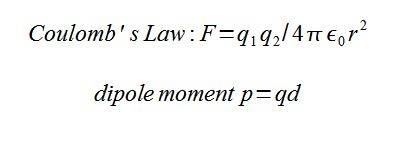# Force between electric dipoles

## Homework Statement

Arrange two electric dipoles with dipole moments qd nose to tail at a distance r (r >> d) along a line including their axis. Use Coulomb's law to show that the force between them varies as r4.

## Homework Equations## The Attempt at a Solution

So in my first attempt, all I did was solve the dipole moment eqn for q, (q1=p1/d, q2=-p2/d) and plug that into Coulomb's law so that you get F= -(p1p2/d2)/(4(pi)(eps0)r2)

Now the part where I am confused is the difference between r and d. If they are just different ways of describing the same thing, then I would get r4 in the denominator which I think then proves that the force falls off at r4.
Is this assumption correct? What is the difference between r and d?

r and d are different .. notice you have (r >> d) in the problem statement.

remember that a dipole consists of two charges separated a small distance.
so the layout looks something like

-q1 ... q1 {space here == distance = r} -q2 ... q2

or as a dipole interaction
--> (d1) {space here == distance = r} --> (d2)
[where d1 = q1*d and d2 = q2*d ]

where the distance d is the distance between -q1 and q1 and
-q2 and q2 and the distance r>>d is the distance between the dipoles.
(might as well consider it the distance from center to center.)

so you'll need to use coulomb's law and some approximation [Taylor's series]
such that r>>d (or d/r << 1).

Ah ok, that makes perfect sense. My textbook author doesn't always break things down that much, but I understand now. Thanks very much for your help!